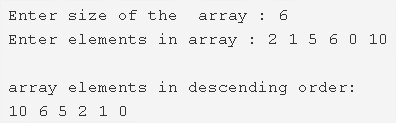# C Program To Sort Array Elements In Descending Order | 3 Ways

C program to sort the array elements in descending order – In this article, we will brief in on the multitude of ways to sort the array elements in a descending order in C programming.

Suitable examples and sample programs have also been added so that you can understand the whole thing very clearly. The compiler has also been added with which you can execute it yourself. If you have any doubts related to C programs to sort the array in descending order leave a comment here.

The ways described in this particular piece are as follows:

• Using Standard Method
• Using Function

As we all know, an array is an arrangement or a sequence of a bunch of elements in any given order whatsoever. The information stored is used in various means in C programming.As you can see in the image uploaded, the size of the array is entered first up.

The size of the array given in this case is 6.

Afterwards, the elements in the array are listed.

The elements in this particular array are 2, 1, 5, 6, 0, 10.

Hence, the descending order of the elements entered is 10, 6, 5, 2, 1, 0.

Thus, the various ways to set a bunch of elements of an array in descending order are as follows:

## Using Standard Method

1. Read the size of the array and store the value into the variable n.

2) scanf reads the entered elements one by one and stores the elements in the array using for loop for(i=0;i<n;i++) as scanf(“%d”&a[i]).

3) Compare the two elements a[j] and a[j+1] and find the least element then swap the elements if a[j] is the least element compare with a[j+1]. The code is as follows

for loop iterates from i=0 to i<n-1

a) for loop iterates from j=0 to j<n-i-1

find the least from a[j] and a[j+1] and swap the two elements, if a[j] is least element than a[j+1].Repeat until all iterations of j.

b) After all iterations of i, the sorted array will be generated in which the elements are in descending order.

4) Print the descending order array elements.

Output:

### Using Function

1. The main() function calls the sort() function by passing array a[],size of the array as arguments.

2) The sort method arranges the array elements in descending order as follows,

a) for loop iterates from i=0 to i<n-1

b) Inner for loop iterates from j=0 to j<n-i-1

Compare adjacent elements a[j] and a[j+1],if a[j] is less then a[j+1] then swap the two elements.Repeat this step    until j<n-i-1.

Repeat the above step until all iterations of i.

3) After all iterations of i, we will get descending order array.

4) The main() calls the print(a,n) to print the descending order array elements.

Output:
x

## C Program To Check If Alphabet, Digit or Special Character | C Programs

C program to check for an alphabet, digit or special character – In this article, ...